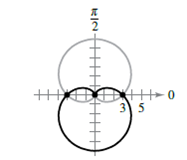Chapter 10.5, Problem 28E

Chapter
Section
Textbook Problem

Finding Points of Intersection In Exercises 27-34, find the points of intersection of the graphs of the equations. r = 3 ( 1 + sin θ ) r = 3 ( 1 − sin θ )To determine

To Find: The point of intersection of graphs.

Explanation

Given:

Formula Used:

r=3(1+sinθ),r=3(1sinθ)

Calculation:

Polar equations are given: r=3(1+sinθ),r=3(1sinθ)

Solve simultaneously and get,

3(1+sinθ)=3(1sinθ)

2sinθ=0

θ=0,π,2π

Substitute θ=0 in r=3(1+sinθ) and get,

r=3(1+sin0)

r=3

Therefore, the point of intersection is (3,0).

Substitute the value of θ=2π in r=3(1+sinθ) and get,

r=3(1+sin2π)

r=3

Therefore, the another point of intersection is (3,2π)

Still sussing out bartleby?

Check out a sample textbook solution.

See a sample solution

The Solution to Your Study Problems

Bartleby provides explanations to thousands of textbook problems written by our experts, many with advanced degrees!

Get Started

Find the numerical value of each expression. 3. (a) cosh(ln 5) (b) cosh 5

Single Variable Calculus: Early Transcendentals, Volume I

Convert the expressions in Exercises 6584 to power form. x2y

Finite Mathematics and Applied Calculus (MindTap Course List)

In O,OY=5 and XZ=6. If XWWY, find WZ.

Elementary Geometry For College Students, 7e

Find the derivative of the function. f(t) = tan(sec(cos t))

Single Variable Calculus: Early Transcendentals

The directrix of the conic given by r=62+10sin is: a) x=53 b) x=35 c) y=53 d) y=35

Study Guide for Stewart's Single Variable Calculus: Early Transcendentals, 8th